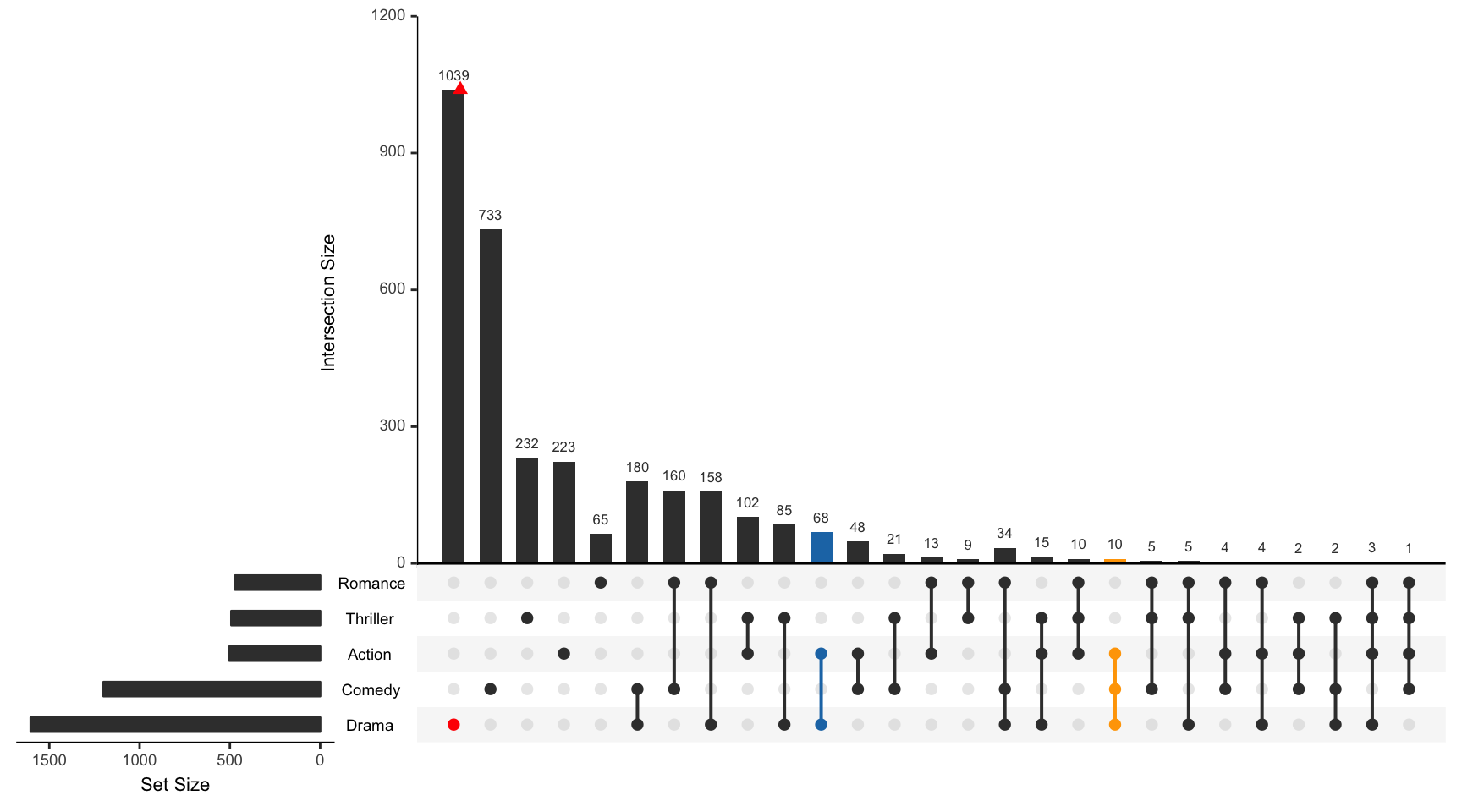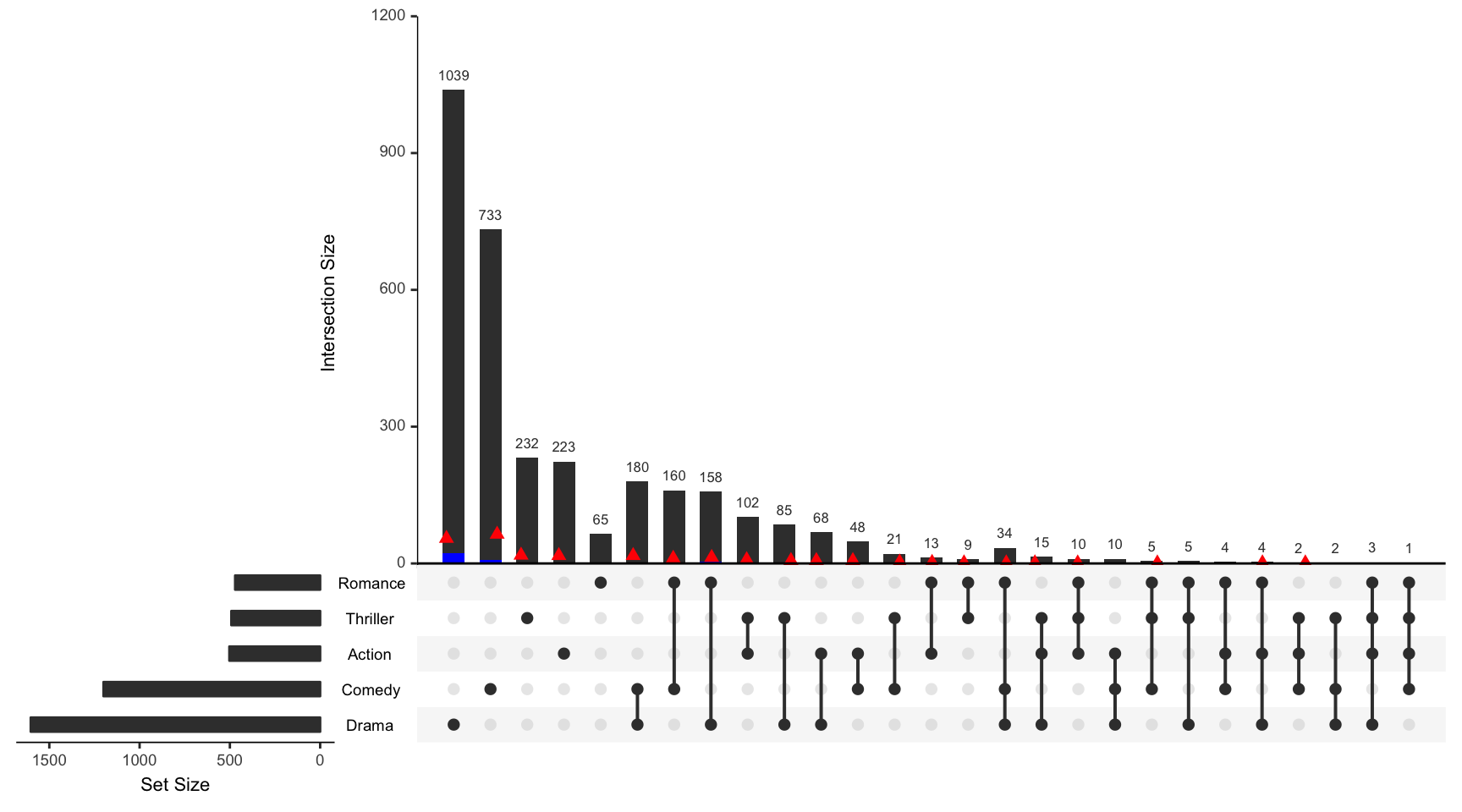For all examples the movies data set contained in the package will be used.

library(UpSetR)
movies <- read.csv(system.file("extdata", "movies.csv", package = "UpSetR"),
header = T, sep = ";")

queries Parameter Breakdown

Each list contained in the queries parameter takes 4 fields: query, params, color, and active.

• query specifies which query is going to be run

• params is a list of paramters for the query to work on

• color is the color that will represent the query on the plot. If no color is provided, a color will be selected from the UpSetR default color palette.

• active determines how the query will be represented on the plot. If active is TRUE, the intersection size bar will be overlayed by a bar representing the query. If active is FALSE, a jitter point will be placed on the intersection size bar.

To learn how queries can be explored and visualized on an element level see the Attribute Plots vignette.

Example 1: Built-in Intersection Query

This example shows how to use the built in intersection query, intersects, to find or display elements in specific intersections. In this example the color selected for the active query is from the default color palette.

upset(movies, queries = list(list(query = intersects, params = list("Drama",
"Comedy", "Action"), color = "orange", active = T), list(query = intersects,
params = list("Drama"), color = "red", active = F), list(query = intersects,
params = list("Action", "Drama"), active = T)))Example 2: Built-In Elements Query

This example shows how to use the built in element query, elements, to visualize how certain elements are distributed amongst the intersections.

upset(movies, queries = list(list(query = elements, params = list("AvgRating",
3.5, 4.1), color = "blue", active = T), list(query = elements, params = list("ReleaseDate",
1980, 1990, 2000), color = "red", active = F)))Example 3: Using Expression Parameter to Subset Intersection and Element Queries

This example shows how to use the expression parameter to subset the results of element and intersection queries.

upset(movies, queries = list(list(query = intersects, params = list("Action",
"Drama"), active = T), list(query = elements, params = list("ReleaseDate",
1980, 1990, 2000), color = "red", active = F)), expression = "AvgRating > 3 & Watches > 100")